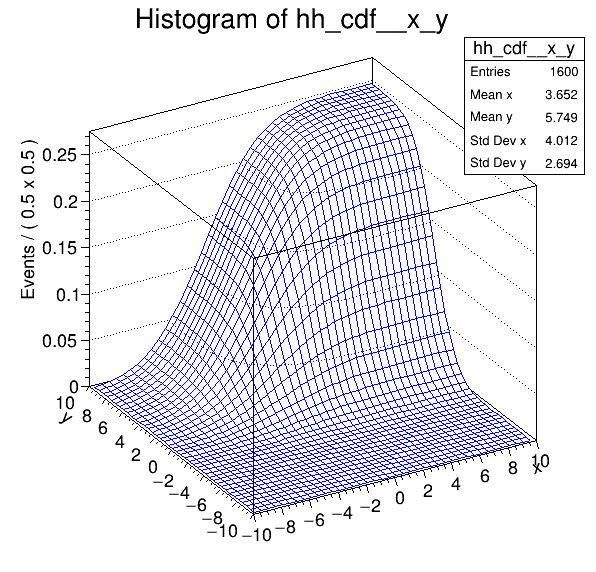# Rf 3 0 8_Normintegration 2D¶

Multidimensional models: normalization and integration of pdfs, construction of cumulative distribution functions from pdfs in two dimensions

Author: Clemens Lange, Wouter Verkerke (C++ version)
This notebook tutorial was automatically generated with ROOTBOOK-izer from the macro found in the ROOT repository on Saturday, November 28, 2020 at 10:46 AM.

In :
from __future__ import print_function
import ROOT

Welcome to JupyROOT 6.23/01


## Set up model¶

Create observables x,y

In :
x = ROOT.RooRealVar("x", "x", -10, 10)
y = ROOT.RooRealVar("y", "y", -10, 10)

RooFit v3.60 -- Developed by Wouter Verkerke and David Kirkby
Copyright (C) 2000-2013 NIKHEF, University of California & Stanford University



Create pdf gaussx(x,-2,3), gaussy(y,2,2)

In :
gx = ROOT.RooGaussian(
"gx", "gx", x, ROOT.RooFit.RooConst(-2), ROOT.RooFit.RooConst(3))
gy = ROOT.RooGaussian(
"gy", "gy", y, ROOT.RooFit.RooConst(+2), ROOT.RooFit.RooConst(2))


gxy = gx(x)*gy(y)

In :
gxy = ROOT.RooProdPdf("gxy", "gxy", ROOT.RooArgList(gx, gy))


## Retrieve raw & normalized values of RooFit pdfs¶

Return 'raw' unnormalized value of gx

In :
print("gxy = ", gxy.getVal())

gxy =  0.4856717852477124


Return value of gxy normalized over x and y in range [-10,10]

In :
nset_xy = ROOT.RooArgSet(x, y)
print("gx_Norm[x,y] = ", gxy.getVal(nset_xy))

gx_Norm[x,y] =  0.012933200957206766


Create object representing integral over gx which is used to calculate gx_Norm[x,y] == gx / gx_Int[x,y]

In :
x_and_y = ROOT.RooArgSet(x, y)
igxy = gxy.createIntegral(x_and_y)
print("gx_Int[x,y] = ", igxy.getVal())

gx_Int[x,y] =  37.552326516436096


NB: it is also possible to do the following

Return value of gxy normalized over x in range [-10,10] (i.e. treating y as parameter)

In :
nset_x = ROOT.RooArgSet(x)
print("gx_Norm[x] = ", gxy.getVal(nset_x))

gx_Norm[x] =  0.1068955044839622


Return value of gxy normalized over y in range [-10,10] (i.e. treating x as parameter)

In :
nset_y = ROOT.RooArgSet(y)
print("gx_Norm[y] = ", gxy.getVal(nset_y))

gx_Norm[y] =  0.12098919425696865


## Integarte normalizes pdf over subrange¶

Define a range named "signal" in x from -5,5

In :
x.setRange("signal", -5, 5)
y.setRange("signal", -3, 3)

[#1] INFO:Eval -- RooRealVar::setRange(x) new range named 'signal' created with bounds [-5,5]
[#1] INFO:Eval -- RooRealVar::setRange(y) new range named 'signal' created with bounds [-3,3]


Create an integral of gxy_Norm[x,y] over x and y in range "signal" ROOT.This is the fraction of of pdf gxy_Norm[x,y] which is in the range named "signal"

In :
igxy_sig = gxy.createIntegral(x_and_y, ROOT.RooFit.NormSet(
x_and_y), ROOT.RooFit.Range("signal"))
print("gx_Int[x,y|signal]_Norm[x,y] = ", igxy_sig.getVal())

gx_Int[x,y|signal]_Norm[x,y] =  0.5720351351990985


## Construct cumulative distribution function from pdf¶

Create the cumulative distribution function of gx i.e. calculate Int[-10,x] gx(x') dx'

In :
gxy_cdf = gxy.createCdf(ROOT.RooArgSet(x, y))


Plot cdf of gx versus x

In :
hh_cdf = gxy_cdf.createHistogram("hh_cdf", x, ROOT.RooFit.Binning(
40), ROOT.RooFit.YVar(y, ROOT.RooFit.Binning(40)))
hh_cdf.SetLineColor(ROOT.kBlue)

c = ROOT.TCanvas("rf308_normintegration2d",
"rf308_normintegration2d", 600, 600)
hh_cdf.GetZaxis().SetTitleOffset(1.8)
hh_cdf.Draw("surf")

c.SaveAs("rf308_normintegration2d.png")

[#0] WARNING:InputArguments -- RooAbsReal::createHistogram(gxy_cdf_Int[x_prime,y_prime|CDF]_Norm[x_prime,y_prime]) WARNING extended mode requested for a non-pdf object, ignored

Info in <TCanvas::Print>: png file rf308_normintegration2d.png has been created


Draw all canvases

In :
from ROOT import gROOT
gROOT.GetListOfCanvases().Draw()